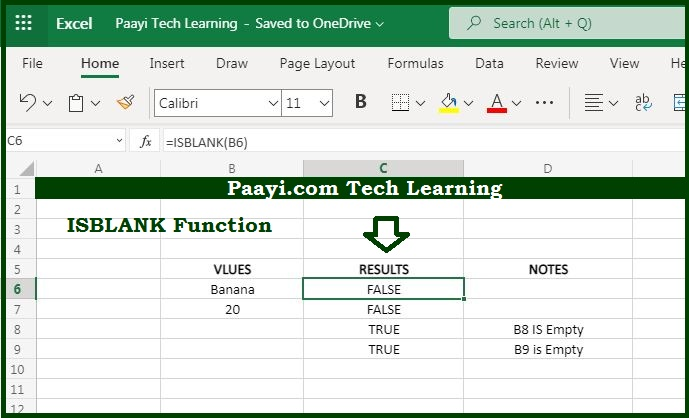# Learn How to Use Microsoft Excel ISBLANK Function

Written by | 0 Comments | 700 Views

In this article, you will learn how to use the Microsoft Excel ISBLANK function and its prime function in Microsoft Excel. You will also get to know the Microsoft Excel ISBLANK function return value and syntax with the help of some examples.

Microsoft Excel ISBLANK Function

The main function of the Microsoft Excel ISBLANK function is to get the information about the cell whether it's blank or not. It implies with the help of the ISBLANK function you can able to get the return value will be TRUE if the particular cell is empty, and FALSE in case it is not empty. Let us understand more about it with the help of the examples given below.

Return Value of ISBLANK Function

The return value will be the logical TRUE or FALSE.

Syntax of ISBLANK Function

=ISBLANK(value)

Where the arguments:

• value: It is the value to check.

## How to Use Microsoft Excel ISBLANK Function?You can use the ISBLANK function to get the information about the cell whether it is empty or not. The ISBLANK will return TRUE if the cell is empty and return FALSE in case the cell is not empty. You can understand this by the examples given in the picture.